# 4.7.6 Natural Polymers (Structured Questions)

Question 1:
Diagram 1.1 shows an experiment to study the effect of vinegar and ammonium solution on latex.
Diagram 1.2 shows the result of the experiment after 30 minutes.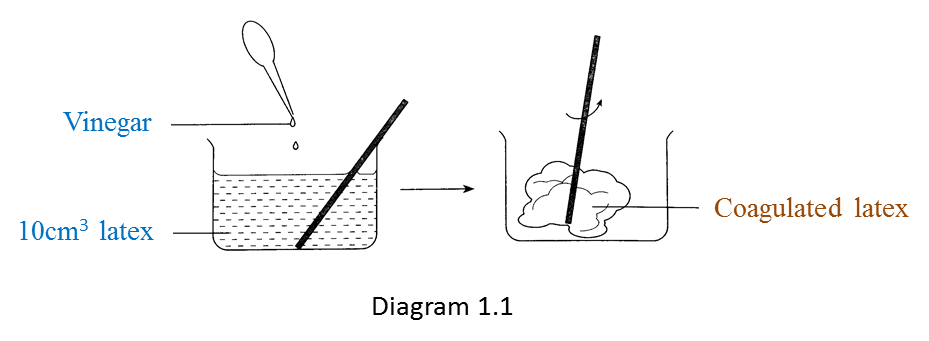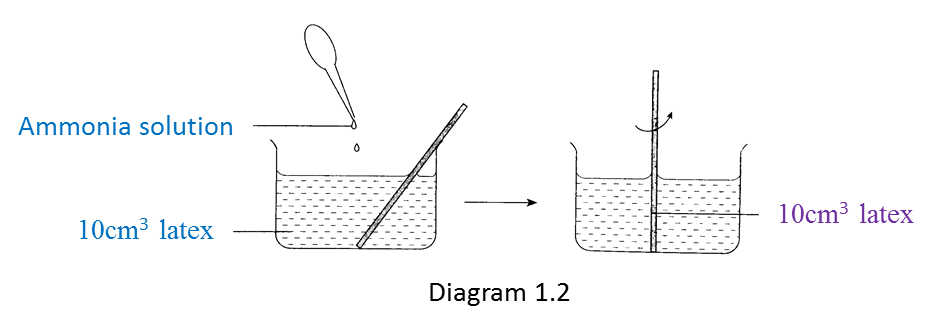(a) Write down one observation from the result of this experiment. [1 mark]

(b) State one inference based on the observation in Diagram 1.1. [1 mark]

(c) State one constant variable in this experiment. [1 mark]

(d) State one hypothesis for this experiment. [1 mark]

(e) Based on this experiment, state the operational definition for acid. [1 mark]

:
(a)
Vinegar causes the coagulation of latex but ammonia solution prevents the coagulation of latex.

(b)
When vinegar (an acid) is mixed with latex, the latex coagulates.
When ammonia solution (an alkali) is mixed with latex, the latex does not coagulate.
(any one)

(c)

1. 10 cm3 of latex
2. Results of the experiment are observed after 30 minutes
(any one)

(d)
Acid causes the coagulation of latex but alkali prevents the coagulation of latex.

(e)
Acid is a substance which causes latex to coagulate.

# 4.7.4 The Oil Palm and Its Importance to National Development (Structured Questions)

Question 1:
Diagram 1.1 shows the process of latex coagulation.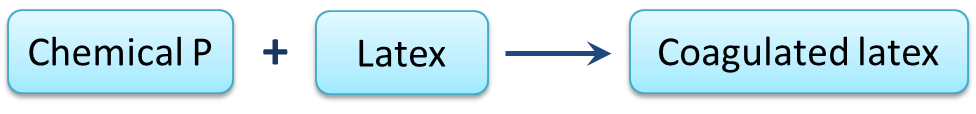(a)(i) State one example of chemical P? [1 mark]

(ii) State one characteristic of coagulated latex in Diagram 1.1. [1 mark]

(b) Chemical P in diagram 1.1 is replaced with chemical Q to prevent latex from coagulating.
State one example of chemical Q. [1 mark]

(c) Diagram 1.2 shows the process when natural rubber is heated with Sulphur to form rubber R.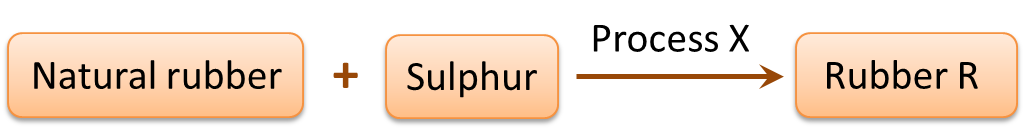(i) Name process X. [1 mark]
(ii) Name rubber R. [1 mark]

(d) Mark (\/) the object which is made of rubber R. [1 mark](a)(i)
Methanoic acid

(a)(ii)
Elastic

(b)
Ammonia solution

(c)(i)
Vulcanization of rubber

(c)(ii)
Vulcanized rubber

(d)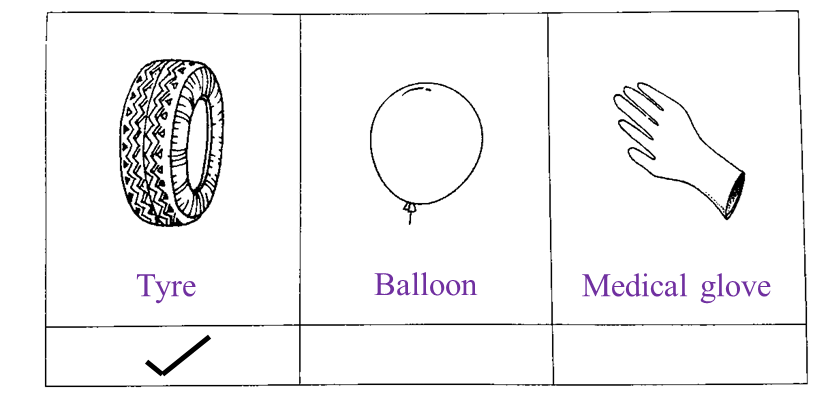# 4.7.2 Alcohol and Its Effects on Health (Structured Questions)

Question 1:
Diagram 1.1 and Diagram 1.2 show an experiment to investigate the effect of temperature on the fermentation of glucose by yeast.(a) State one hypothesis that can be made from this experiment. [1 mark]

(b) State the variables in this experiment.
(i) Manipulated variable [1 mark]
(ii) Responding variable [1 mark]

(c) Based on Diagram 1.1 and 1.2, which temperature is more suitable for the fermentation of glucose? [1 mark]

(d) Diagram 1.3 shows the graph of the volume of carbon dioxide produced at 35oC against time.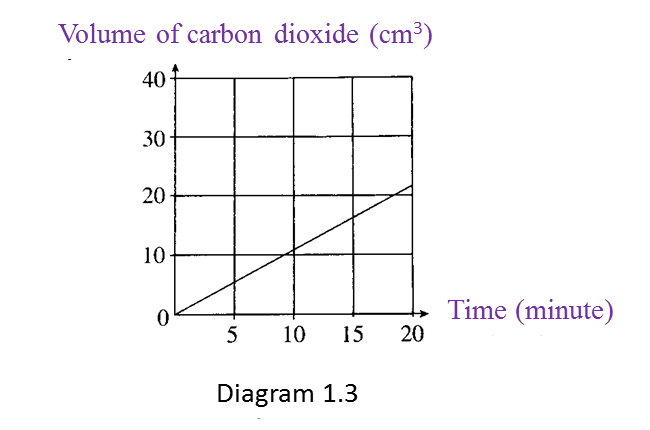What is the relationship between the volume of carbon dioxide produced and time? [1 mark]

(a) Fermentation of glucose by yeast is affected by temperature.

(b)(i)
Temperature of the water bath

(b)(ii)
Volume of carbon dioxide produced

(c)
35oC

(d)
The volume of carbon dioxide produced is directly proportional to time.

# 4.7.1 Various Carbon Compound (Structured Questions)

Question 1:
Diagram 1.1 shows two examples of carbon compounds, K and L.(a)(i) Based on Diagram 1.1, which one is an inorganic carbon compound? [1 mark]

(ii) State one characteristic of an inorganic carbon compound. [1 mark]

(b)(i) State one use of compound K. [1 mark]

(ii) State one effect of compound K on the nervous system if consumed excessively. [1 mark]

(c) Diagram 1.2 shows a tank containing gas M used for gas stoves.
Gas is a hydrocarbon compound.(i) State two elements present in gas M. [1 mark]

(ii) State one source of gas M. [1 mark]

(a)(i) L or marble chips

(a)(ii)
Originates from non-living things or does not originates from living things

(b)(i)
Alcoholic drink

(b)(ii)
Disrupts nerve coordination or slows down the transmission of impulses

(c)(i)
1. Hydrogen
2. Carbon

(c)(ii)
Petroleum